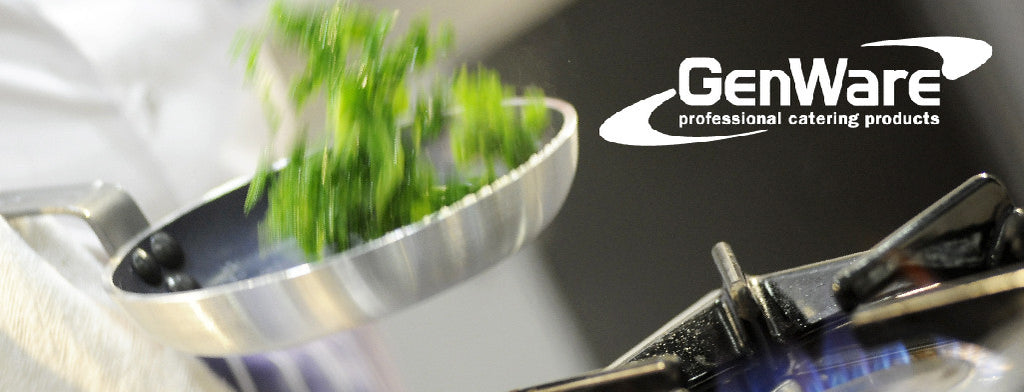Shopping Cart

### Refine

View all

#### Price# Genware£4.30 each (ex VAT)£73.80 each (ex VAT)£8.19 each (ex VAT)£3.71 each (ex VAT)£7.68 each (ex VAT)£2.50 each (ex VAT)£5.95 each (ex VAT)£1.14 each (ex VAT)£10.58 each (ex VAT)£43.98 each (ex VAT)£8.19 each (ex VAT)£49.22 each (ex VAT)£11.08 each (ex VAT)£20.66 each (ex VAT)£30.02 each (ex VAT)£13.98 each (ex VAT)£17.64 each (ex VAT)£24.32 each (ex VAT)£15.90 each (ex VAT)£14.64 each (ex VAT)£18.12 each (ex VAT)£17.12 each (ex VAT)£54.12 each (ex VAT)£67.42 each (ex VAT)£99.18 each (ex VAT)£126.12 each (ex VAT)£5.71 each (ex VAT)£3.95 each (ex VAT)£4.12 each (ex VAT)£4.24 each (ex VAT)£5.20 each (ex VAT)£3.71 each (ex VAT)£3.50 each (ex VAT)£6.28 each (ex VAT)£14.50 each (ex VAT)£2.68 each (ex VAT)£15.88 each (ex VAT)£28.32 each (ex VAT)£23.78 each (ex VAT)£3.40 each (ex VAT)£31.00 each (ex VAT)£37.18 each (ex VAT)£24.80 each (ex VAT)£7.66 each (ex VAT)£38.78 each (ex VAT)£15.88 each (ex VAT)£20.42 each (ex VAT)£24.56 each (ex VAT)